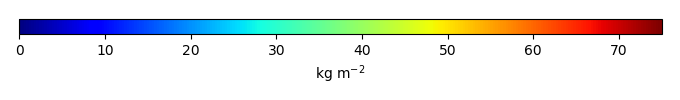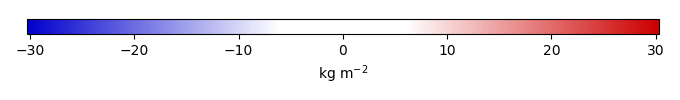# Mean State

Period Mean (original grids) [Pg]
Model Period Mean (intersection) [Pg]
Model Period Mean (complement) [Pg]
Benchmark Period Mean (intersection) [Pg]
Benchmark Period Mean (complement) [Pg]
Bias [kg m-2]
Bias Score 
Spatial Distribution Score 
Overall Score 
Benchmark [-] 34.1
CLM5PHSOFF [-] 33.5 32.8 1.04 33.8 0.315 -0.225 0.71 0.94 0.82
CLM5PHSON [-] 30.5 30.9 0.269 33.8 0.315 -0.226 0.73 0.95 0.84
Period Mean (original grids) [Pg]
Model Period Mean (intersection) [Pg]
Model Period Mean (complement) [Pg]
Benchmark Period Mean (intersection) [Pg]
Benchmark Period Mean (complement) [Pg]
Bias [kg m-2]
Bias Score 
Spatial Distribution Score 
Overall Score 
Benchmark [-] 44.8
CLM5PHSOFF [-] 14.4 13.7 0.0504 44.4 0.478 -4.51 0.50 0.64 0.57
CLM5PHSON [-] 15.3 14.4 0.0241 44.4 0.478 -4.37 0.50 0.78 0.64
Period Mean (original grids) [Pg]
Model Period Mean (intersection) [Pg]
Model Period Mean (complement) [Pg]
Benchmark Period Mean (intersection) [Pg]
Benchmark Period Mean (complement) [Pg]
Bias [kg m-2]
Bias Score 
Spatial Distribution Score 
Overall Score 
Benchmark [-] 60.1
CLM5PHSOFF [-] 31.2 27.7 2.83 59.3 0.762 -3.34 0.54 0.84 0.69
CLM5PHSON [-] 28.7 27.8 0.749 59.3 0.762 -2.94 0.55 0.84 0.69
Period Mean (original grids) [Pg]
Model Period Mean (intersection) [Pg]
Model Period Mean (complement) [Pg]
Benchmark Period Mean (intersection) [Pg]
Benchmark Period Mean (complement) [Pg]
Bias [kg m-2]
Bias Score 
Spatial Distribution Score 
Overall Score 
Benchmark [-] 49.3
CLM5PHSOFF [-] 66.9 64.9 2.40 48.3 0.997 4.73 0.47 0.27 0.37
CLM5PHSON [-] 101. 99.2 1.16 48.3 0.997 17.5 0.43 0.12 0.28
Period Mean (original grids) [Pg]
Model Period Mean (intersection) [Pg]
Model Period Mean (complement) [Pg]
Benchmark Period Mean (intersection) [Pg]
Benchmark Period Mean (complement) [Pg]
Bias [kg m-2]
Bias Score 
Spatial Distribution Score 
Overall Score 
Benchmark [-] 55.7
CLM5PHSOFF [-] 41.7 33.9 6.36 50.7 4.99 -4.94 0.68 0.30 0.49
CLM5PHSON [-] 20.6 19.1 1.31 50.7 4.99 -6.12 0.65 0.27 0.46
Period Mean (original grids) [Pg]
Model Period Mean (intersection) [Pg]
Model Period Mean (complement) [Pg]
Benchmark Period Mean (intersection) [Pg]
Benchmark Period Mean (complement) [Pg]
Bias [kg m-2]
Bias Score 
Spatial Distribution Score 
Overall Score 
Benchmark [-] 131.
CLM5PHSOFF [-] 74.8 72.0 0.825 130. 0.462 -6.50 0.60 0.88 0.74
CLM5PHSON [-] 133. 130. 0.561 130. 0.462 0.0856 0.64 0.88 0.76
Period Mean (original grids) [Pg]
Model Period Mean (intersection) [Pg]
Model Period Mean (complement) [Pg]
Benchmark Period Mean (intersection) [Pg]
Benchmark Period Mean (complement) [Pg]
Bias [kg m-2]
Bias Score 
Spatial Distribution Score 
Overall Score 
Benchmark [-] 153.
CLM5PHSOFF [-] 63.0 62.2 2.04 151. 2.09 -4.82 0.54 0.84 0.69
CLM5PHSON [-] 91.8 93.6 0.579 151. 2.09 -2.83 0.62 0.90 0.76
Period Mean (original grids) [Pg]
Model Period Mean (intersection) [Pg]
Model Period Mean (complement) [Pg]
Benchmark Period Mean (intersection) [Pg]
Benchmark Period Mean (complement) [Pg]
Bias [kg m-2]
Bias Score 
Spatial Distribution Score 
Overall Score 
Benchmark [-] 58.4
CLM5PHSOFF [-] 36.8 32.2 3.01 56.8 1.57 -3.42 0.60 0.82 0.71
CLM5PHSON [-] 33.0 31.1 0.855 56.8 1.57 -2.67 0.66 0.86 0.76
Period Mean (original grids) [Pg]
Model Period Mean (intersection) [Pg]
Model Period Mean (complement) [Pg]
Benchmark Period Mean (intersection) [Pg]
Benchmark Period Mean (complement) [Pg]
Bias [kg m-2]
Bias Score 
Spatial Distribution Score 
Overall Score 
Benchmark [-] 59.4
CLM5PHSOFF [-] 87.0 86.3 1.69 58.9 0.523 7.71 0.50 0.47 0.48
CLM5PHSON [-] 119. 120. 0.656 58.9 0.523 18.1 0.45 0.23 0.34
Period Mean (original grids) [Pg]
Model Period Mean (intersection) [Pg]
Model Period Mean (complement) [Pg]
Benchmark Period Mean (intersection) [Pg]
Benchmark Period Mean (complement) [Pg]
Bias [kg m-2]
Bias Score 
Spatial Distribution Score 
Overall Score 
Benchmark [-] 58.9
CLM5PHSOFF [-] 31.3 26.4 4.46 57.4 1.53 -3.60 0.53 0.63 0.58
CLM5PHSON [-] 27.6 25.8 1.60 57.4 1.53 -2.75 0.54 0.47 0.51
Period Mean (original grids) [Pg]
Model Period Mean (intersection) [Pg]
Model Period Mean (complement) [Pg]
Benchmark Period Mean (intersection) [Pg]
Benchmark Period Mean (complement) [Pg]
Bias [kg m-2]
Bias Score 
Spatial Distribution Score 
Overall Score 
Benchmark [-] 66.2
CLM5PHSOFF [-] 39.5 36.0 2.67 64.6 1.58 -4.96 0.63 0.86 0.75
CLM5PHSON [-] 44.2 42.7 0.830 64.6 1.58 -2.44 0.72 0.85 0.78
Period Mean (original grids) [Pg]
Model Period Mean (intersection) [Pg]
Model Period Mean (complement) [Pg]
Benchmark Period Mean (intersection) [Pg]
Benchmark Period Mean (complement) [Pg]
Bias [kg m-2]
Bias Score 
Spatial Distribution Score 
Overall Score 
Benchmark [-] 1.38e+03
CLM5PHSOFF [-] 864. 814. 72.1 1.32e+03 56.4 -3.60 0.57 0.27 0.42
CLM5PHSON [-] 1.06e+03 1.04e+03 16.5 1.32e+03 56.4 -1.32 0.60 0.43 0.51
Period Mean (original grids) [Pg]
Model Period Mean (intersection) [Pg]
Model Period Mean (complement) [Pg]
Benchmark Period Mean (intersection) [Pg]
Benchmark Period Mean (complement) [Pg]
Bias [kg m-2]
Bias Score 
Spatial Distribution Score 
Overall Score 
Benchmark [-] 64.3
CLM5PHSOFF [-] 7.36 6.25 1.08 63.6 0.726 -3.51 0.41 0.61 0.51
CLM5PHSON [-] 14.0 13.8 0.386 63.6 0.726 -2.91 0.48 0.82 0.65
Period Mean (original grids) [Pg]
Model Period Mean (intersection) [Pg]
Model Period Mean (complement) [Pg]
Benchmark Period Mean (intersection) [Pg]
Benchmark Period Mean (complement) [Pg]
Bias [kg m-2]
Bias Score 
Spatial Distribution Score 
Overall Score 
Benchmark [-] 90.9
CLM5PHSOFF [-] 73.1 68.9 3.63 89.5 1.44 -2.89 0.52 0.60 0.56
CLM5PHSON [-] 123. 122. 1.25 89.5 1.44 5.76 0.44 0.42 0.43
Period Mean (original grids) [Pg]
Model Period Mean (intersection) [Pg]
Model Period Mean (complement) [Pg]
Benchmark Period Mean (intersection) [Pg]
Benchmark Period Mean (complement) [Pg]
Bias [kg m-2]
Bias Score 
Spatial Distribution Score 
Overall Score 
Benchmark [-] 42.5
CLM5PHSOFF [-] 23.2 19.6 2.87 40.2 2.26 -6.41 0.56 0.68 0.62
CLM5PHSON [-] 16.9 15.5 0.942 40.2 2.26 -5.56 0.57 0.73 0.65
Period Mean (original grids) [Pg]
Model Period Mean (intersection) [Pg]
Model Period Mean (complement) [Pg]
Benchmark Period Mean (intersection) [Pg]
Benchmark Period Mean (complement) [Pg]
Bias [kg m-2]
Bias Score 
Spatial Distribution Score 
Overall Score 
Benchmark [-] 45.4
CLM5PHSOFF [-] 20.3 18.7 0.872 44.5 0.888 -6.20 0.58 0.58 0.58
CLM5PHSON [-] 32.2 31.2 0.308 44.5 0.888 -2.77 0.72 0.77 0.75
Period Mean (original grids) [Pg]
Model Period Mean (intersection) [Pg]
Model Period Mean (complement) [Pg]
Benchmark Period Mean (intersection) [Pg]
Benchmark Period Mean (complement) [Pg]
Bias [kg m-2]
Bias Score 
Spatial Distribution Score 
Overall Score 
Benchmark [-] 88.1
CLM5PHSOFF [-] 45.7 45.5 0.638 86.3 1.76 -7.70 0.55 0.68 0.62
CLM5PHSON [-] 75.2 75.3 0.272 86.3 1.76 -1.69 0.63 0.87 0.75
Period Mean (original grids) [Pg]
Model Period Mean (intersection) [Pg]
Model Period Mean (complement) [Pg]
Benchmark Period Mean (intersection) [Pg]
Benchmark Period Mean (complement) [Pg]
Bias [kg m-2]
Bias Score 
Spatial Distribution Score 
Overall Score 
Benchmark [-] 34.9
CLM5PHSOFF [-] 7.06 7.30 0.325 34.7 0.216 -6.86 0.46 0.73 0.59
CLM5PHSON [-] 11.5 12.1 0.0886 34.7 0.216 -5.41 0.54 0.87 0.71
Period Mean (original grids) [Pg]
Model Period Mean (intersection) [Pg]
Model Period Mean (complement) [Pg]
Benchmark Period Mean (intersection) [Pg]
Benchmark Period Mean (complement) [Pg]
Bias [kg m-2]
Bias Score 
Spatial Distribution Score 
Overall Score 
Benchmark [-] 101.
CLM5PHSOFF [-] 74.2 72.2 1.62 100. 0.811 -3.26 0.69 0.52 0.60
CLM5PHSON [-] 68.5 67.8 0.372 100. 0.811 -3.35 0.69 0.46 0.58
Period Mean (original grids) [Pg]
Model Period Mean (intersection) [Pg]
Model Period Mean (complement) [Pg]
Benchmark Period Mean (intersection) [Pg]
Benchmark Period Mean (complement) [Pg]
Bias [kg m-2]
Bias Score 
Spatial Distribution Score 
Overall Score 
Benchmark [-] 60.7
CLM5PHSOFF [-] 41.4 40.3 1.47 60.4 0.300 -2.91 0.65 0.76 0.70
CLM5PHSON [-] 47.2 47.5 0.479 60.4 0.300 -1.44 0.67 0.84 0.75
Period Mean (original grids) [Pg]
Model Period Mean (intersection) [Pg]
Model Period Mean (complement) [Pg]
Benchmark Period Mean (intersection) [Pg]
Benchmark Period Mean (complement) [Pg]
Bias [kg m-2]
Bias Score 
Spatial Distribution Score 
Overall Score 
Benchmark [-] 29.6
CLM5PHSOFF [-] 16.2 14.1 1.51 28.2 1.46 -4.22 0.52 0.54 0.53
CLM5PHSON [-] 15.7 14.8 0.272 28.2 1.46 -3.23 0.57 0.49 0.53

# Temporally integrated period mean

BENCHMARK MEANMODEL MEANBIASBIAS SCORESPATIAL TAYLOR DIAGRAMMODEL COLORS# Spatially integrated regional mean

MODEL COLORS# All Models

BenchmarkCLM5PHSOFFCLM5PHSON# Data Information

Approach: I just read the data from the original data file and flip the latitude arrange from original 90N->90S to 90S->90N.

Temporal resolution: yearly

General information: This product was derived from Harmonized World Soil Database (HWSD) v1.2 dataset.

Spatial resolution: 0.5x0.5 degree